# 669 and Level 4

Since all its digits are divisible by 3, obviously 669 is divisible by 3.

222 + 223 + 224 = 669. Thus 669 is the sum of 3 consecutive numbers. Prime factor 223 is the middle number in the sum.

Also consecutive numbers 109 + 110 + 111 + 112 + 113 + 114 = 669Print the puzzles or type the solution on this excel file: 12 Factors 2015-11-02

—————————————————————————————————

• 669 is a composite number.
• Prime factorization: 669 = 3 x 223
• The exponents in the prime factorization are 1 and 1. Adding one to each and multiplying we get (1 + 1)(1 + 1) = 2 x 2 = 4. Therefore 669 has exactly 4 factors.
• Factors of 669: 1, 3, 223, 669
• Factor pairs: 669 = 1 x 669 or 3 x 223
• 669 has no square factors that allow its square root to be simplified. √669 ≈ 25.865034.—————————————————————————————————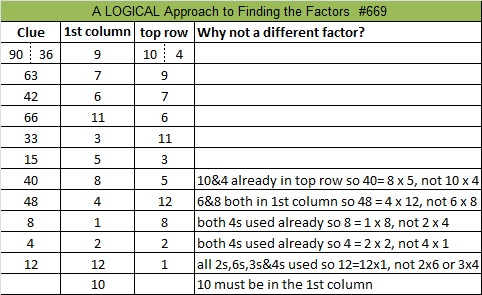Ricardo shows the solution here:

//platform.twitter.com/widgets.js

# 329 and A Last Minute Gift

• 329 is a composite number.
• Prime factorization: 329 = 7 x 47
• The exponents in the prime factorization are 1 and 1. Adding one to each and multiplying we get (1 + 1)(1 + 1) = 2 x 2 = 4. Therefore 329 has exactly 4 factors.
• Factors of 329: 1, 7, 47, 329
• Factor pairs: 329 = 1 x 329 or 7 x 47
• 329 has no square factors that allow its square root to be simplified. √329 ≈ 18.138Here’s a puzzle that could be a last minute gift. If there is a child in you life who has recently become familiar with the multiplication table, put this puzzle in his or her stocking! It is as easy as the puzzles get.Print or type on this week’s puzzles using this excel file: 10 Factors 2014-12-22Last year on Christmas Eve I offered a free last minute gift, a puzzle booklet, but I discovered that most people take care of their last minute gift-giving a couple of days sooner than that. Still this last minute gift could be sent electronically and you can’t beat the price.

I also learned that margins in excel can move so the booklet didn’t always print up as nicely as I wanted. I’m still recovering from surgery so this year I simply revised that same booklet.

I saved the booklet as Factor Holiday pdf to eliminate those printing issues.  In pdf, the lines on the puzzles became quite dark so I don’t like the way they look as much, but I’ll live with it.

The booklet is also available in Factor Holiday excel if you prefer to type your answers directly on the computer. You can try printing it off of excel as well, but I can’t guarantee what the margins will do at any given time.

Here’s a copy of the puzzle booklet’s cover with my sincere holiday greetings for all of you: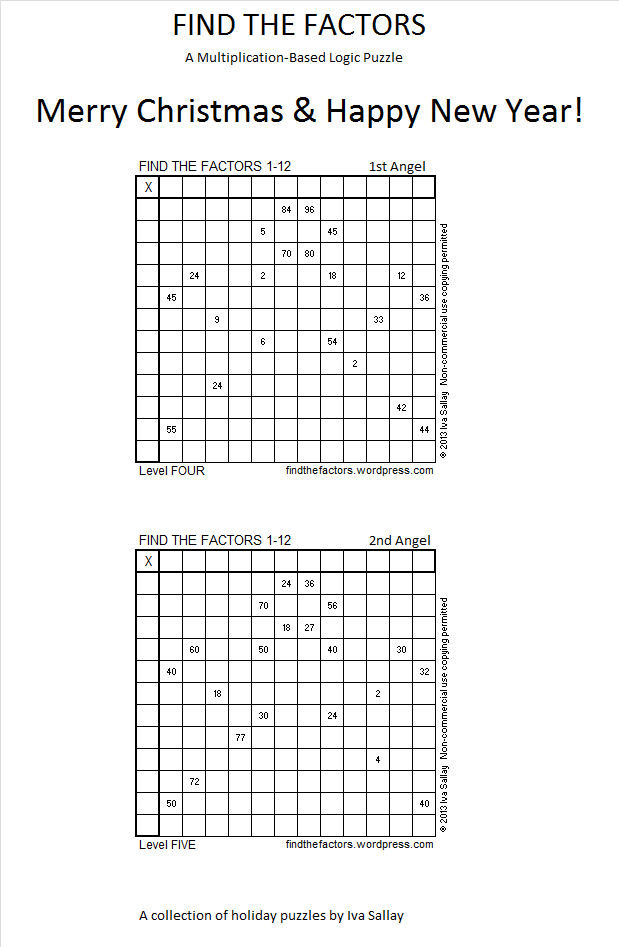# 72 and Level 4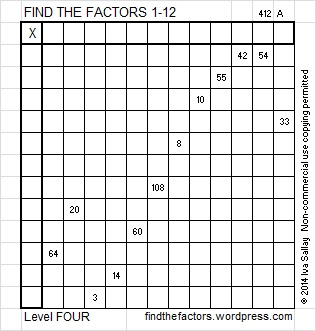This week’s puzzles for you to solve

Here are some facts about the number 72:

72 is a composite number. 72 = 1 x 72, 2 x 36, 3 x 24, 4 x 18, 6 x 12, or 8 x 9. Factors of 72: 1, 2, 3, 4, 6, 8, 9, 12, 18, 24, 36, 72. Prime factorization: 72 = 2 x 2 x 2 x 3 x 3, which can also be written 2³ x 3²Sometimes 72 is a clue in the Find the Factors puzzles. Even though it has many other factors, we use only 8 x 9 for the FIND THE FACTORS 1-10 puzzles and 8 x 9 or 6 x 12 for the FIND THE FACTORS 1-12 puzzles.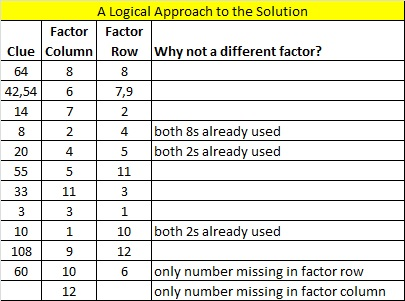# 68 and Happy Saint Patrick’s Day!

68 is a composite number. 68 = 1 x 68, 2 x 34, or 4 x 17. Factors of 68: 1, 2, 4, 17, 34, 68. Prime factorization: 68 = 2 x 2 x 17, which can also be written 2² x 17.68 is never a clue in the FIND THE FACTORS puzzles.All Previously Published Puzzles

I survived my daughter’s wedding. The last of our out-of-state visitors will be going home tomorrow. AND my computer that broke and spent a week in the shop is working again. I will publish six new puzzles next Monday.# 64 and Can You See the Logic Here?

64 is a composite number, and it is 8 squared. 64 = 1 x 64, 2 x 32, 4 x 16, or 8 x 8. Factors of 64: 1, 2, 4, 8, 16, 32, 64. Prime factorization: 64 = 2 x 2 x 2 x 2 x 2 x 2, which can also be written 64 = 2⁶.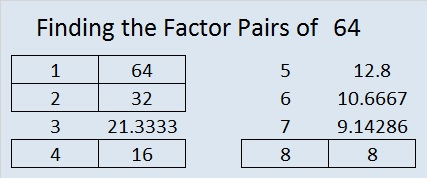Since √64 = 8, a whole number, 64 is a perfect square.

Sometimes 64 is a clue in the FIND THE FACTORS puzzles. Even though it has other factors, when it is a clue, the factors are always 8 x 8.

64 is in this cool pattern: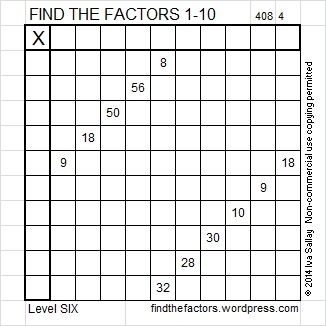All Previously Published PuzzlesThis solution is complicated, but here is a logical explanation:

There are only two 3s available, so at most one 9 can be 3 x 3.

Can both 9s be 9 x 1? If both were, then both 1s would be used, leaving 8 = 2 x 4 and 10 = 2 x 5 as the only possibilities for 8 and 10. But if both 9s were 9 x 1, then the 18 in the same row as the 9 would have to be 18 = 9 x 2, and that would use too many twos. Therefore, EXACTLY ONE of the nines can use 9 = 9 x 1.

Since one 9 = 3 x 3, using both 3s, 30 must equal 5 x 6. And since both 30 and 50 must use both 5s, 10 must equal 10 x 1.

Since 10 = 10 x 1 and a 9 = 9 x 1, 8 must equal 2 x 4. So now finally we can begin filling out the puzzle:# 63 and Twelve Quick Clues

63 is a composite number. 63 = 1 x 63, 3 x 21, or 7 x 9. Factors of 63: 1, 3, 7, 9, 21, 63. Prime factorization: 63 = 3 x 3 x 7, which can also be written 63 = 3² x 7.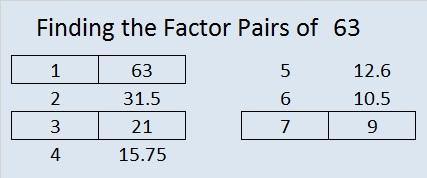Sometimes 63 is a clue in the FIND THE FACTORS puzzles. Even though it has other factors, the only multiplication fact we use is 7 x 9 = 63.All Previously Published Puzzles# 62 and Love: Challenging But Worth It

62 is a composite number. 62 = 1 x 62 or 2 x 31. Factors of 62: 1, 2, 31, 62. Prime factorization: 62 = 2 x 31.62 is never a clue in the FIND THE FACTORS puzzles.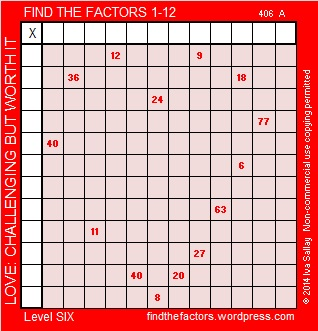All Previously Published PuzzlesHere is the logic to solve the puzzle & a chart showing one way to get the solution:

9 has to be the factor of both the 27 and the 63.  Therefore 3 x 6 = 18, (not 2 x 9).

3 is one of the factors of both the 18 and the 27.  Therefore 9 x 1 = 9, (not 3 x 3).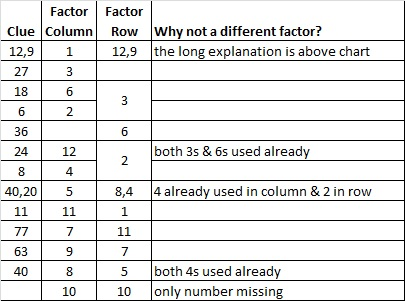# 61 and Opportunity to Catch Up

• 61 is a prime number.
• Prime factorization: 61 is prime.
• The exponent of prime number 61 is 1. Adding 1 to that exponent we get (1 + 1) = 2. Therefore 61 has exactly 2 factors.
• Factors of 61: 1, 61
• Factor pairs: 61 = 1 x 61
• 61 has no square factors that allow its square root to be simplified. √61 ≈ 7.8102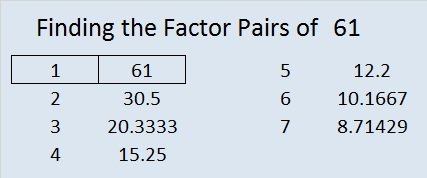How do we know that 61 is a prime number? If 61 were not a prime number, then it would be divisible by at least one prime number less than or equal to √61 ≈ 7.8. Since 61 cannot be divided evenly by 2, 3, 5, or 7, we know that 61 is a prime number.

61 is never a clue in the FIND THE FACTORS puzzles.

My daughter is getting married in early March, and family will be coming in from out of town. I also need to study for a test at work. There is so much that needs to get done that hasn’t been getting done. I’m taking a month-long break from daily blogging and a few other things so I can catch up on the things that just can’t wait.

Over the last several weeks there has been several new people who have found this blog and have done some of the puzzles. Some of them have clicked on the older excel files to work on puzzles. All that clicking was probably inconvenient, but several people have still clicked anyway. Thank you for your interest and persistence. I want to make it easier to catch up on all those old puzzles.

To make it easier to access the older puzzles, I’ve put all previously published FIND THE FACTORS 1-10 and 1-12 puzzles in one excel file, All Previously Published Puzzles. The formatting isn’t printer friendly, but if you enable editing, you can type the factors directly onto the excel file. The answers are also in the file for easy comparison with your work. (Hopefully, I didn’t mess up any of the clues while I hurriedly created this large file. Please, tell me if you find any errors.)While I catch up on all the things I need to do, I hope you will catch up a few things as well.

# 60 and Pay No Attention to That Man Behind the Curtain

### Today’s Puzzle:

When solving a level 6 puzzle, trying to place the numbers from 1 to 10 in the top row as well as the first column can be frustrating. Every single clue appears to have more than one possible answer. How can someone guess right with every single number placement? Is seeing the answer the only way to solve the puzzle?

Here is this week’s level 6 puzzle. Usually, to solve a puzzle, you start with a row or column with 2 clues.  There are 6 rows or columns with more than one clue, and every one of them is no help!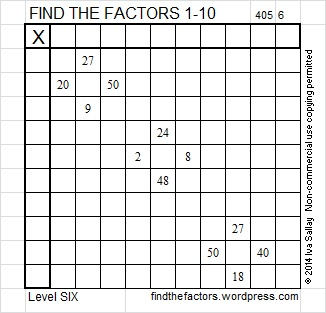You can also find this and a few other puzzles in an excel file here. If you have a spreadsheet program on your computer, you can access it. If you enable editing in excel, you can type your answers directly onto the puzzle, and you can also easily print the puzzles.

### A Peek at the Possible Factors for Each of Last Week’s Clues:

“Pay no attention to the that man behind the curtain!” is a famous line from The Wizard of Oz.  Everyone in Oz thought the Wizard was powerful enough to solve any problem. When Dorthy and her friends looked behind the curtain they discovered that he was really an ordinary man who was able to find an advantage in what other people didn’t know. It turns out that other people actually had the abilities they thought only the Wizard had. Today we will take a peek behind the curtain of a level 6 puzzle and discover that it really isn’t as intimidating as we might imagine and that you have the ability to solve such a puzzle, too. This is what last week’s level 6 puzzle looks like from behind the curtain:Many people try to start with the row that contains the clue 4 x 8 = 32. (When I solve this puzzle, that row is actually the one I FINISH with!) Instead, look at the row with no clues. If you study all the clues in the puzzle, you will notice that 6 is listed as a factor only once. If there were a clue in the row without any clues, that clue would be a multiple of 6. That hint will keep you going for several clues. It also eliminates 8 as a possible factor of 72 and ensures that 1 x 8, not 2 x 4, must be used for the clue 8. This means that 2 x 5, not 1 x 10, must be used for the clue 10 because both ones will have been used. That means that 2 x 10, not 4 x 5, must be used for the clue 20 because the number 10 has to be used as a factor twice. Here is the completed puzzle:### Factors of 60:

60 is a composite number. 60 = 1 x 60, 2 x 30, 3 x 20, 4 x 15, 5 x 12, or 6 x 10. Factors of 60: 1, 2, 3, 4, 5, 6, 10, 12, 15, 20, 30, 60. Prime factorization: 60 = 2 x 2 x 3 x 5, which can also be written 60 = 2² x 3 x 5.Sometimes 60 is a clue in the FIND THE FACTORS puzzles. Even though it has many other factors, we only use 6 x 10 for the FIND THE FACTORS 1-10 puzzles and 6 x 10 or 5 x 12 for the FIND THE FACTORS 1-12 puzzles.

### Sum-Difference Puzzle:

60 has six factor pairs. One of those factor pairs adds up to 17, and another one subtracts to 17. If you can identify those factor pairs, then you can solve this puzzle: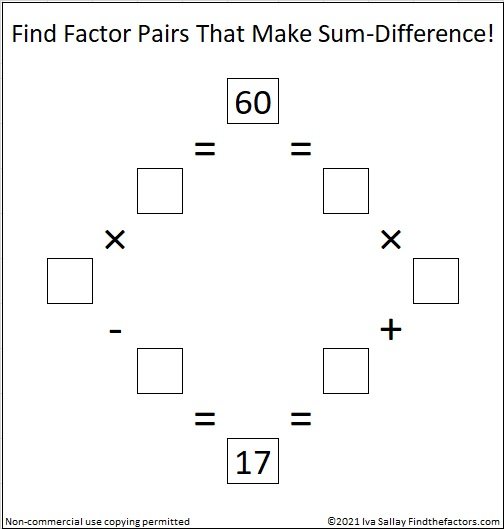# 59 and Valentine Gif

• 59 is a prime number.
• Prime factorization: 59 is prime.
• The exponent of prime number 59 is 1. Adding 1 to that exponent we get (1 + 1) = 2. Therefore 59 has exactly 2 factors.
• Factors of 59: 1, 59
• Factor pairs: 59 = 1 x 59
• 59 has no square factors that allow its square root to be simplified. √59 ≈ 7.6811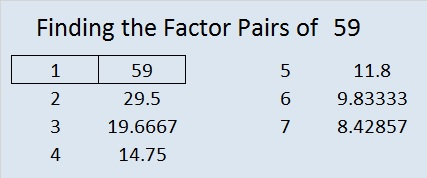How do we know that 59 is a prime number? If 59 were not a prime number, then it would be divisible by at least one prime number less than or equal to √59 ≈ 7.7. Since 59 cannot be divided evenly by 2, 3, 5, or 7, we know that 59 is a prime number.

59 is never a clue in the FIND THE FACTORS puzzles.Valentine’s Day is almost here. Here is a Valentine you can share with your friends who enjoy solving puzzles.

To solve the puzzle, the numbers 1 through 10 need to be written on the top row and also on the first column so that those numbers and the clues inside the puzzle make a multiplication table. Since it’s a level 5 puzzle, it may be a little challenging.

Your friend will not have to wait a whole week for the solution because there is a gif that will solve it quickly and logically. The gif could be the valentine, too.

This week’s puzzles are available in an excel file here. If you have a spreadsheet program on your computer, you can access it. If you enable editing in excel, you can type your answers directly onto the puzzle, and you can also easily print the puzzles.

Here are the factors to last week’s puzzle.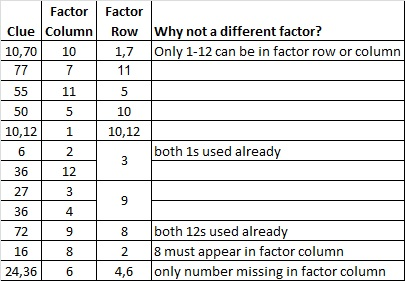<a data-pin-do=”embedPin” href=”http://www.pinterest.com/pin/506092076847813688/”></a&gt;
<!– Please call pinit.js only once per page –>
//assets.pinterest.com/js/pinit.js